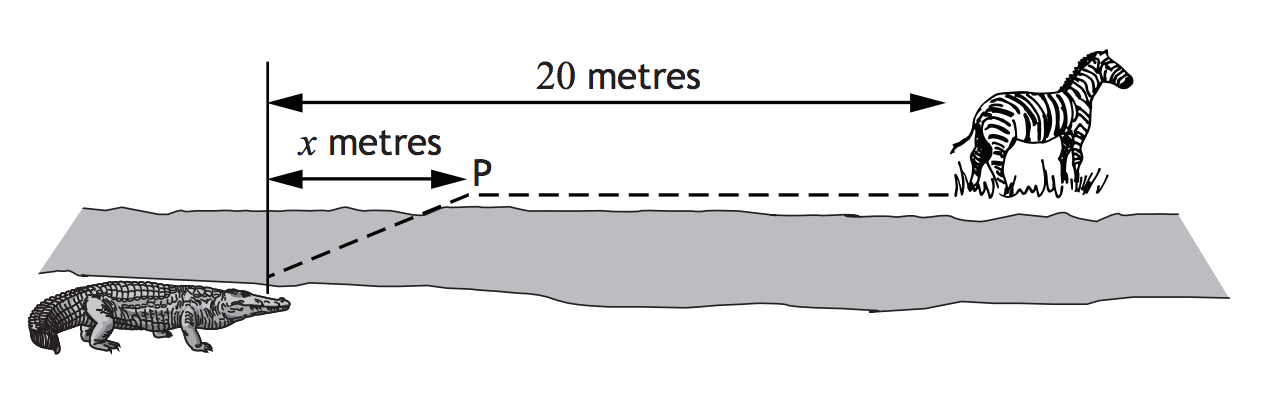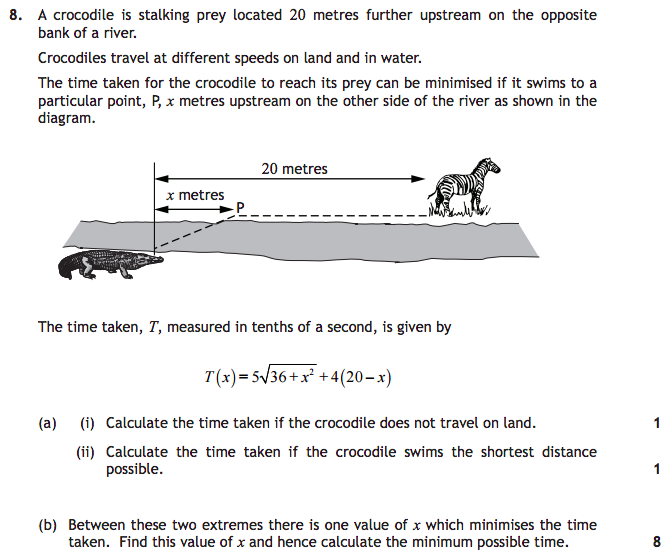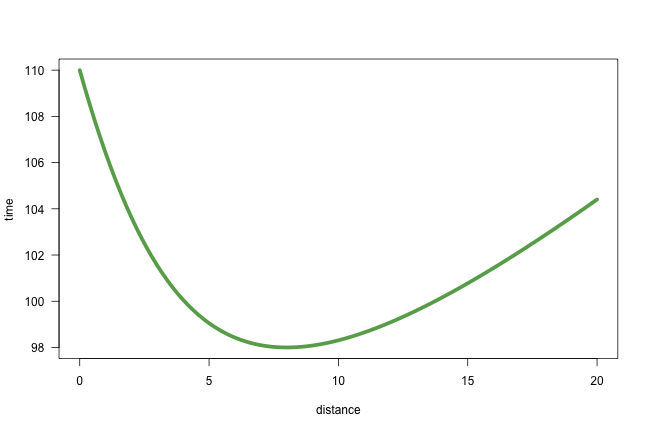# Solving the Crocodile Math Problem in R

##### Posted on October 19, 2015

Using R to solve the crocodile math problem from the Scottish Higher Maths exam of May 2015.### Motivation

Reading the Spanish newspaper La Vanguardia, I found a curious article about a math problem that S4 Scottish students (the equivalent of high school’s senior year) struggled with last May on their SQA Higher Maths test.

Here’s the problem.### Getting Started

The first thing to do is write a function containing the formula of $$T(x)$$ the time taken for the crocodile to reach its prey:

Now let’s look at this function. I’ll choose an interval of (0, 20) for $$x$$ since this represents the distance (0 meters, 20 meters).### First question

Calculate the time taken if the crocodile does not travel on land. That means that the crocodile swims the entire 20 meters.

### Second question

Calculate the time taken if the crocodile swims the shortest distance possible. Personally I think that this question is poorly written and/or bad designed. What does the “shortest distance possible” mean? Assuming that the shortest distance possible is 0 meters, then the time taken to swim 0 meters is:

### Third question

Between these two extremes there is one value of $$x$$ which minimizes the time taken. Find this value of $$x$$ and hence calculate the minimum possible time. This is a typical calculus problem of minimizing a function. In this case the function to be minimized is the time taken. This implies calculating the derivative of $$T(x)$$.

We can use the function deriv() to compute the derivative of a simple expression (symbolically).

As you can tell from the output of deriv(), the fifth line contains the desired derivative: .grad[, "x"] <- 5 * (0.5 * (2 * x * .expr2^-0.5)) - 4

We can also obtain this derivative by using the function D() and pass it the body of the function croctime() like so:

Looking at the graphic of time, the curve has a minimum somewhere between 7 meters and 10 meters. We can evaluate the derivative g() with some x values to try to narrow down our search for the minimum value.

Aha! the minimum value is at a distance of 8 meters! To confirm that 8 is actually the root of the polynomial, we can use the function multiroot() from the package "rootSolve" by Karline Soetaert

There you go: the minimum taken time for the crocodile to catch the zebra is given when the crocodile swims 8 meters, and then walks the other 12 meters.

Published in categories how-to  Tagged with crocodile